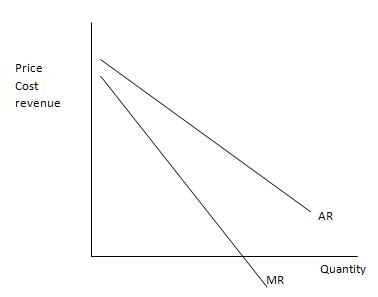### Monopolist’s Demand and MR Curve

A monopolist will have a negative demand curve.Being a Monopolist , the firm can keep its prices as high as it wants. AR curve is the Demand curve for the firm.A monopoly firm will have a negative AR and MR curves.
Monopoly’s Revenue
• Total Revenue = P x Q = TR
• Average Revenue = TR/Q = AR = P
• Marginal Revenue = DTR/DQ = MR

Monopoly’s Marginal Revenue
• A monopolist’s marginal revenue is always less than the price of its good.
• The demand curve is downward sloping.
• When a monopoly drops the price to sell one more unit, the revenue received from previously sold units also decreases.
• When a monopoly increases the amount it sells, it has two effects on total revenue (P x Q).
• The output effect—more output is sold, so Q is higher.
• The price effect—price falls, so P is lower.